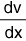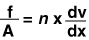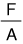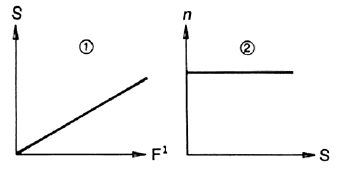### Viscosity

The viscosity of a fluid is that property which tends to resist a shearing force. It can be thought of as the internal friction resulting when one layer of fluid is made to move in relation to another layer.

Consider the model shown in Fig. 1, which was used by Isaac Newton in first defining viscosity. It shows two parallel planes of fluid of area A separated by a distance dx and moving in the same direction at different velocities V1 and V2.Fig. 1
The velocity distribution will be linear over the distance dx, and experiments show that the velocity gradient,is directly proportional to the force per unit area,Where n constant for a given liquid and is called its viscosity.

The velocity gradient,describes the shearing experienced by the intermediate layers as they move with respect to each other. Therefore, it can be called the "rate of shear", S. Also, the force per unit area,can be simplified and called the "shear force" or "shear stress," F. With these simplified terms, viscosity can be defined as follows'.Isaac Newton made the assumption that all materials have, at a given temperature, a viscosity that is independent of the rate of shear. In other words, a force twice as large would be required to move a liquid twice as fast. Fluids which behave this way are called Newtonian fluids. There are, of course, fluids which do not behave this way, in other words their viscosity is dependent on the rate of shear. These are known as Non-Newtonian fluids.

Fig. 2 shows graphically the relationships between shear Stress (F,) rate of shear (S,) and viscosity (n) for a Newtonian liquid. The viscosity remains constant as shown in sketch 2, and in absolute units, the viscosity is the inverse slope of the line in sketch 1. Water and light oils are good examples of Newtonian liquids.Fig. 2 Newtonian Liquid